# 交叉熵Loss¶

$L=-\sum_{c=1}^My_clog(p_c)$

#二值交叉熵，这里输入要经过sigmoid处理
import torch
import torch.nn as nn
import torch.nn.functional as F
nn.BCELoss(F.sigmoid(input), target)
#多分类交叉熵, 用这个 loss 前面不需要加 Softmax 层
nn.CrossEntropyLoss(input, target)


# 带权交叉熵 Loss¶

$L=-\sum_{c=1}^Mw_cy_clog(p_c)$

$w_c=\frac{N-N_c}{N}$

# Focal Loss¶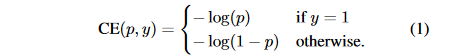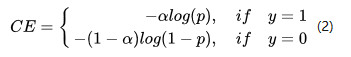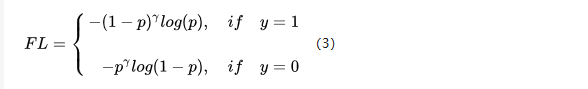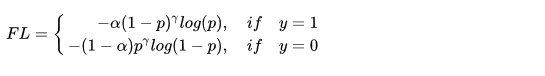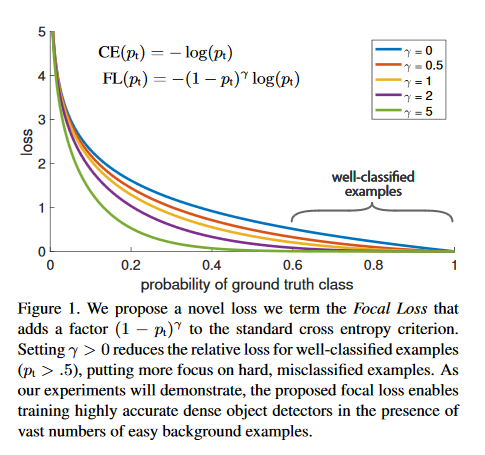Focal Loss的Pytorch代码实现如下：

class FocalLoss(nn.Module):
def __init__(self, gamma=0, alpha=None, size_average=True):
super(FocalLoss, self).__init__()
self.gamma = gamma
self.alpha = alpha
if isinstance(alpha,(float,int,long)): self.alpha = torch.Tensor([alpha,1-alpha])
if isinstance(alpha,list): self.alpha = torch.Tensor(alpha)
self.size_average = size_average

def forward(self, input, target):
if input.dim()>2:
input = input.view(input.size(0),input.size(1),-1)  # N,C,H,W => N,C,H*W
input = input.transpose(1,2)    # N,C,H*W => N,H*W,C
input = input.contiguous().view(-1,input.size(2))   # N,H*W,C => N*H*W,C
target = target.view(-1,1)

logpt = F.log_softmax(input)
logpt = logpt.gather(1,target)
logpt = logpt.view(-1)
pt = Variable(logpt.data.exp())

if self.alpha is not None:
if self.alpha.type()!=input.data.type():
self.alpha = self.alpha.type_as(input.data)
at = self.alpha.gather(0,target.data.view(-1))
logpt = logpt * Variable(at)

loss = -1 * (1-pt)**self.gamma * logpt
if self.size_average: return loss.mean()
else: return loss.sum()


# Dice Loss¶

• Dice系数：根据 Lee Raymond Dice命令，是用来度量集合相似度的度量函数，通常用于计算两个样本之间的像素，公式如下：

$s=\frac{2|X\bigcap Y|}{|X|+|Y|}$

$s=\frac{2TP}{2TP+FN+FP}$

• Dice Loss：公式定义为 ：

$loss = 1 - \frac{2|X\bigcap Y|}{|X|+|Y|}$

Dice Loss使用与样本极度不均衡的情况，如果一般情况下使用Dice Loss会回反向传播有不利的影响，使得训练不稳定。

• 训练分割网络，例如FCN，UNet是选择交叉熵Loss还是选择Dice Loss？

• 假设我们用$p$来表示预测值，而$t$来表示真实标签值，那么交叉熵损失关于$p$的梯度形式类似于$p-t$（我会在文后给出推导），而Dice Loss的值是$1- \frac{2pt}{p^2+t^2}$$1 - \frac{2pt}{p+t}$，其关于$p$的梯度形式为$\frac{2t^2}{(p+t)^2}$$\frac{2t(t^2-p^2)}{(p^2+t^2)^2}$，可以看到在极端情况下即$p$$t$都很小的时候，计算得到的梯度值可能会非常大，即会导致训练十分不稳定。
• 另外一种解决类别不平衡的方法是简单的对每一个类别根据赋予不同的权重因子（如对数量少的类别赋予较大的权重因子），使得样本数量不均衡问题得到缓解（上面已经介绍过了，就是带权重的交叉熵Loss）。
• 这两种处理方式，哪种更好，还是建议自己针对自己的数据做一个实验。
• 代码实现：
import torch.nn as nn
import torch.nn.functional as F

class SoftDiceLoss(nn.Module):
def __init__(self, weight=None, size_average=True):
super(SoftDiceLoss, self).__init__()

def forward(self, logits, targets):
num = targets.size(0)
// 为了防止除0的发生
smooth = 1

probs = F.sigmoid(logits)
m1 = probs.view(num, -1)
m2 = targets.view(num, -1)
intersection = (m1 * m2)

score = 2. * (intersection.sum(1) + smooth) / (m1.sum(1) + m2.sum(1) + smooth)
score = 1 - score.sum() / num
return score


# IOU Loss¶

IOU Loss和Dice Loss一样属于metric learning的衡量方式，公式定义如下：

$L = 1 - \frac{A\bigcap B}{A \bigcup B}$ 它和Dice Loss一样仍然存在训练过程不稳定的问题，IOU Loss在分割任务中应该是不怎么用的，如果你要试试的话代码实现非常简单，在上面Dice Loss的基础上改一下分母部分即可，不再赘述了。我们可以看一下将IOU loss应用到FCN上在VOC 2010上的实验结果：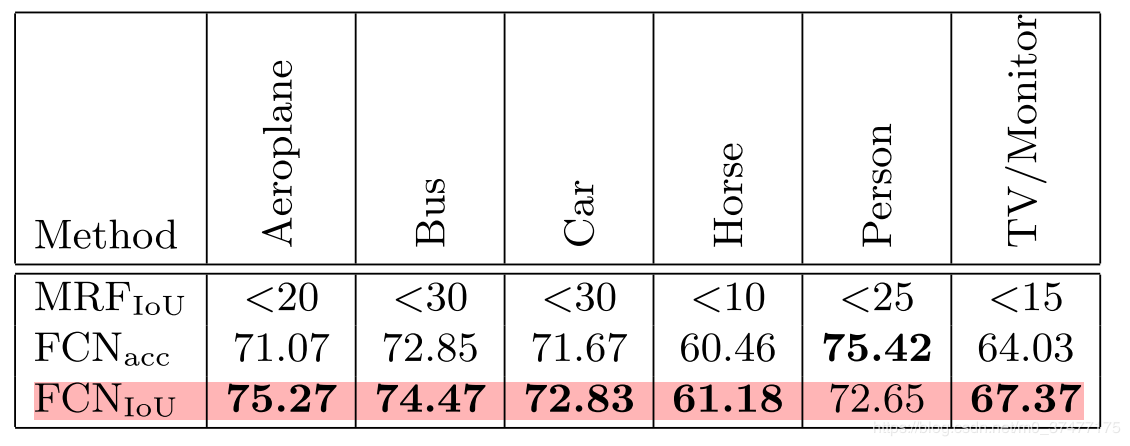# Tversky Loss¶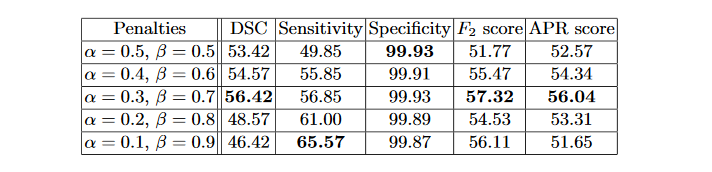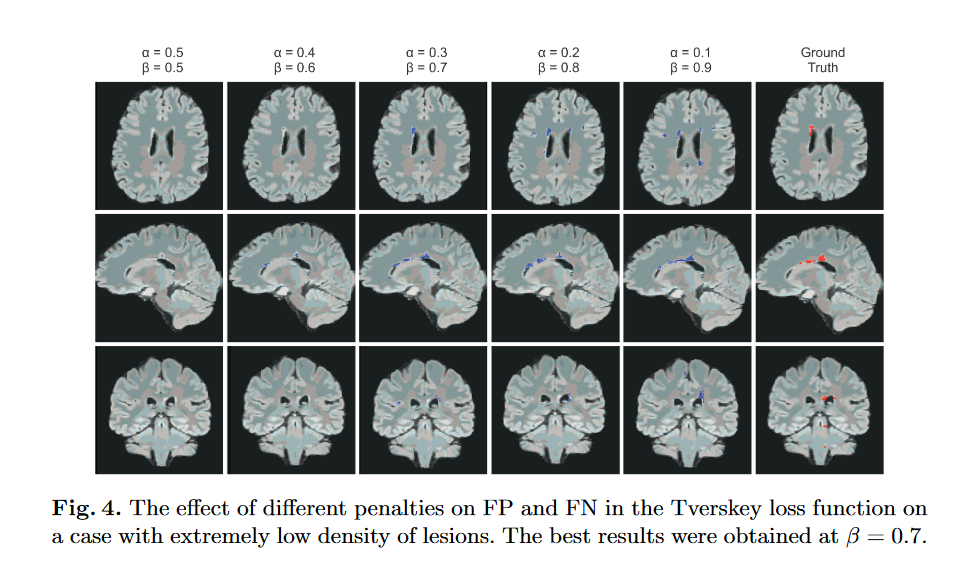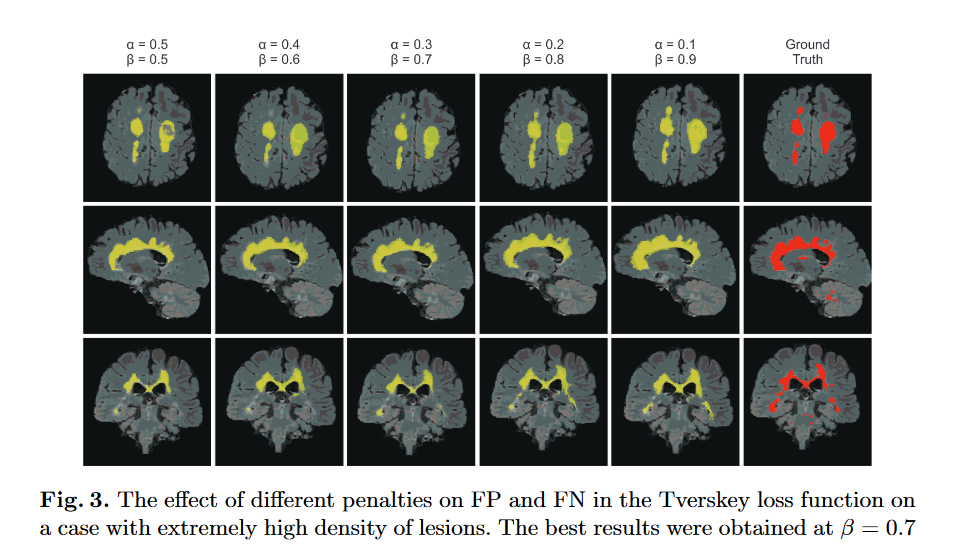Keras代码实现如下：

def tversky(y_true, y_pred):
y_true_pos = K.flatten(y_true)
y_pred_pos = K.flatten(y_pred)
true_pos = K.sum(y_true_pos * y_pred_pos)
false_neg = K.sum(y_true_pos * (1-y_pred_pos))
false_pos = K.sum((1-y_true_pos)*y_pred_pos)
alpha = 0.7
return (true_pos + smooth)/(true_pos + alpha*false_neg + (1-alpha)*false_pos + smooth)

def tversky_loss(y_true, y_pred):
return 1 - tversky(y_true,y_pred)


# Generalized Dice loss¶

Keras代码实现：

def generalized_dice_coeff(y_true, y_pred):
Ncl = y_pred.shape[-1]
w = K.zeros(shape=(Ncl,))
w = K.sum(y_true, axis=(0,1,2))
w = 1/(w**2+0.000001)
# Compute gen dice coef:
numerator = y_true*y_pred
numerator = w*K.sum(numerator,(0,1,2,3))
numerator = K.sum(numerator)
denominator = y_true+y_pred
denominator = w*K.sum(denominator,(0,1,2,3))
denominator = K.sum(denominator)
gen_dice_coef = 2*numerator/denominator
return gen_dice_coef
def generalized_dice_loss(y_true, y_pred):
return 1 - generalized_dice_coeff(y_true, y_pred)


# Focal Loss + Dice Loss¶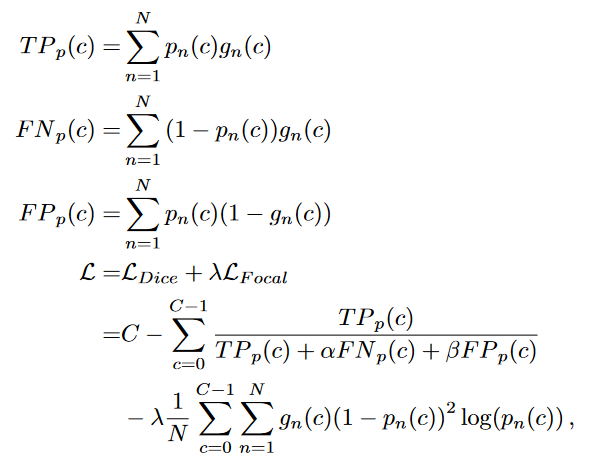# Exponential Logarithmic loss¶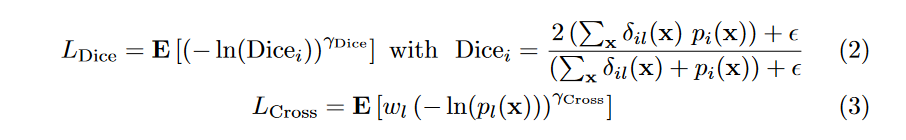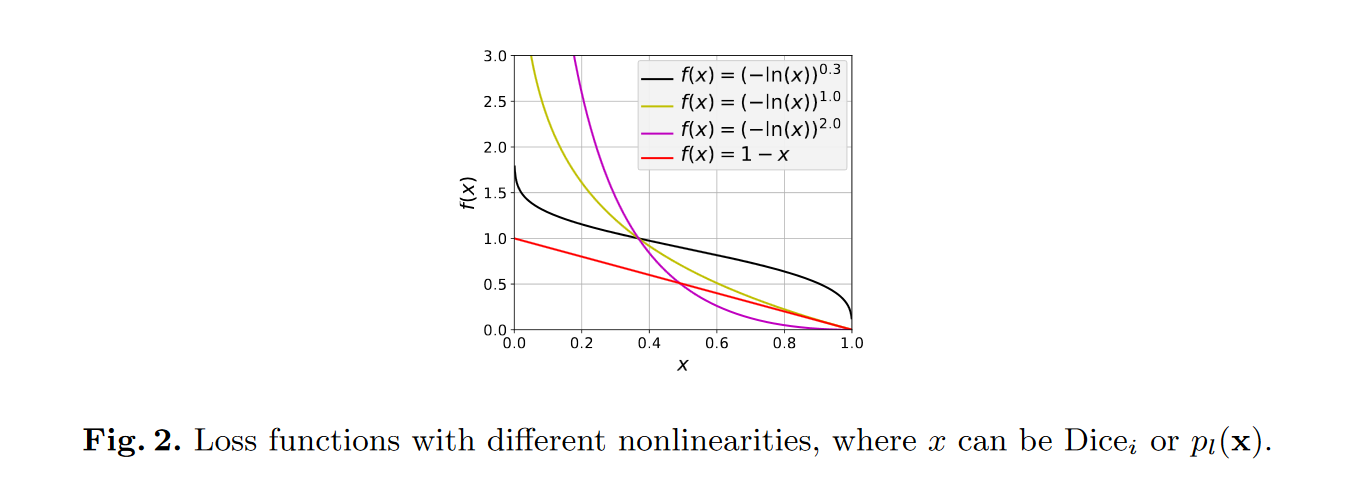# 补充（Softmax梯度计算）¶

(1)softmax函数 $\quad$首先再来明确一下softmax函数，一般softmax函数是用来做分类任务的输出层。softmax的形式为: $S_i = \frac{e^{z_i}}{\sum_ke^{z_k}}$ 其中$S_i$表示的是第i个神经元的输出，接下来我们定义一个有多个输入，一个输出的神经元。神经元的输出为 $z_i = \sum_{ij}x_{ij}+b$ 其中$w_{ij}$是第$i$个神经元的第$j$个权重,b是偏移值.$z_i$表示网络的第$i$个输出。给这个输出加上一个softmax函数，可以写成: $a_i = \frac{e^{z_i}}{\sum_ke^{z_k}}$, 其中$a_i$表示softmax函数的第i个输出值。这个过程可以用下图表示: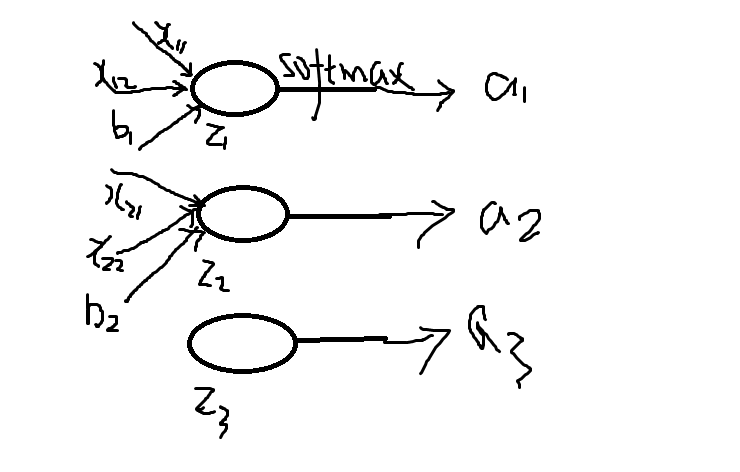(2)损失函数 softmax的损失函数一般是选择交叉熵损失函数，交叉熵函数形式为： $C=-\sum_i{y_i lna_i}$ 其中y_i表示真实的标签值 (3)需要用到的高数的求导公式

c'=0(c为常数）
(x^a)'=ax^(a-1),a为常数且a≠0
(a^x)'=a^xlna
(e^x)'=e^x
(logax)'=1/(xlna),a>0且 a≠1
(lnx)'=1/x
(sinx)'=cosx
(cosx)'=-sinx
(tanx)'=(secx)^2
(secx)'=secxtanx
(cotx)'=-(cscx)^2
(cscx)'=-csxcotx
(arcsinx)'=1/√(1-x^2)
(arccosx)'=-1/√(1-x^2)
(arctanx)'=1/(1+x^2)
(arccotx)'=-1/(1+x^2)
(shx)'=chx
(chx)'=shx
（uv)'=uv'+u'v
(u+v)'=u'+v'
(u/)'=(u'v-uv')/^2

(3)进行推导 我们需要求的是loss对于神经元输出$z_i$的梯度，求出梯度后才可以反向传播，即是求: $\frac{\partial C}{\partial z_i}$, 根据链式法则(也就是复合函数求导法则)$\frac{\partial C}{\partial a_j}\frac{\partial a_j}{\partial z_i}$，初学的时候这个公式理解了很久，为什么这里是$a_j$而不是$a_i$呢？这里我们回忆一下softmax的公示，分母部分包含了所有神经元的输出，所以对于所有输出非i的输出中也包含了$z_i$，所以所有的a都要参与计算，之后我们会看到计算需要分为$i=j$$i \neq j$两种情况分别求导数。 首先来求前半部分：

$\frac{\partial C}{ \partial a_j} = \frac{-\sum_jy_ilna_j}{\partial a_j} = -\sum_jy_j\frac{1}{a_j}$

• 如果$i=j$$\frac{\partial a_i}{\partial z_i} = \frac{\partial(\frac{e^{z_i}}{\sum_ke^{z_k}})}{\partial z_i}=\frac{\sum_ke^{z_k}e^{z_i}-(e^{z_i})^2}{(\sum_ke^{z_k})^2}=(\frac{e^z_i}{\sum_ke^{z_k}})(1 - \frac{e^{z_i}}{\sum_ke^{z_k}})=a_i(1-a_i)$
• 如果$i \neq j$$\frac{\partial a_i}{\partial z_i}=\frac{\partial\frac{e^{z_j}}{\sum_ke^{z_k}}}{\partial z_i} = -e^{z_j}(\frac{1}{\sum_ke^z_k})^2e^{z_i}=-a_ia_j$

$\frac{\partial C}{\partial z_i}$ $=(-\sum_{j}y_j\frac{1}{a_j})\frac{\partial a_j}{\partial z_i}$ $=-\frac{y_i}{a_i}a_i(1-a_i)+\sum_{j \neq i}\frac{y_j}{a_j}a_ia_j$ $=-y_i+y_ia_i+\sum_{j \neq i}\frac{y_j}a_i$ $=-y_i+a_i\sum_{j}y_j$

$\frac{\partial C}{\partial z_i}=a_i - y_i$

#coding=utf-8
import numpy as np

def softmax_loss_native(W, X, y, reg):
'''
Softmax_loss的暴力实现，利用了for循环
输入的维度是D，有C个分类类别，并且我们在有N个例子的batch上进行操作
输入:
- W: 一个numpy array，形状是(D, C)，代表权重
- X: 一个形状为(N, D)为numpy array，代表输入数据
- y: 一个形状为(N,)的numpy array，代表类别标签
- reg: (float)正则化参数
f返回:
- 一个浮点数代表Loss
- 和W形状一样的梯度
'''
loss = 0.0
dW = np.zeros_like(W) #dW代表W反向传播的梯度
num_classes = W.shape
num_train = X.shape
for i in xrange(num_train):
scores = X[i].dot(W)
shift_scores = scores - max(scores) #防止数值不稳定
loss_i = -shift_scores[y[i]] + np.log(sum(np.exp(shift_scores)))
loss += loss_i
for j in xrange(num_classes):
softmax_output = np.exp(shift_scores[j]) / sum(np.exp(shift_scores))
if j == y[i]:
dW[:, j] += (-1 + softmax_output) * X[i]
else:
dW[:, j] += softmax_output * X[i]
loss /= num_train
loss += 0.5 * reg * np.sum(W * W)
dW = dW / num_train + reg * W
return loss, dW

def softmax_loss_vectorized(W, X, y, reg):
loss = 0.0
dW = np.zeros_like(W)
num_class = W.shape
num_train = X.shape
scores = X.dot(W)
shift_scores = scores - np.max(scores, axis=1).reshape(-1, 1)
softmax_output  = np.exp(shift_scores) / np.sum(np.exp(shift_scores), axis=1).reshape(-1, 1)
loss = -np.sum(np.log(softmax_output[range(num_train), list(y)]))
loss /= num_train
loss += 0.5 * reg * np.sum(W * W)
dS = softmax_output.copy()
dS[range(num_train), list(y)] += -1
dW = (x.T).dot(dS)
dW = dW/num_train + reg*W
return loss, dW


# 推荐阅读¶